xCyclonic separationEncyclopedia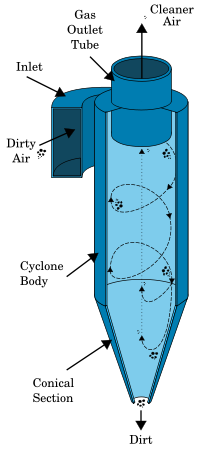Cyclonic separation is a method of removing particulates from an air, gas or liquid stream, without the use of filters, through vortex
Vortex
A vortex is a spinning, often turbulent,flow of fluid. Any spiral motion with closed streamlines is vortex flow. The motion of the fluid swirling rapidly around a center is called a vortex...

separation. Rotation
Rotation
A rotation is a circular movement of an object around a center of rotation. A three-dimensional object rotates always around an imaginary line called a rotation axis. If the axis is within the body, and passes through its center of mass the body is said to rotate upon itself, or spin. A rotation...

al effects and gravity are used to separate mixtures of solids and fluids. The method can also be used to separate fine droplets of liquid from a gaseous stream.

A high speed rotating (air)flow is established within a cylindrical or conical container called a cyclone. Air flows in a helical pattern, beginning at the top (wide end) of the cyclone and ending at the bottom (narrow) end before exiting the cyclone in a straight stream through the center of the cyclone and out the top. Larger (denser) particles in the rotating stream have too much inertia to follow the tight curve of the stream, and strike the outside wall, then falling to the bottom of the cyclone where they can be removed. In a conical system, as the rotating flow moves towards the narrow end of the cyclone, the rotational radius of the stream is reduced, thus separating smaller and smaller particles. The cyclone geometry, together with flow rate, defines the cut point of the cyclone. This is the size of particle that will be removed from the stream with a 50% efficiency. Particles larger than the cut point will be removed with a greater efficiency, and smaller particles with a lower efficiency.

An alternative cyclone design uses a secondary air flow within the cyclone to keep the collected particles from striking the walls, to protect them from abrasion. The primary air flow containing the particulates enters from the bottom of the cyclone and is forced into spiral rotation by stationary spinner vanes. The secondary air flow enters from the top of the cyclone and moves downward toward the bottom, intercepting the particulate from the primary air. The secondary air flow also allows the collector to optionally be mounted horizontally, because it pushes the particulate toward the collection area, and does not rely solely on gravity to perform this function.

Large scale cyclones are used in sawmill
Sawmill
A sawmill is a facility where logs are cut into boards.-Sawmill process:A sawmill's basic operation is much like those of hundreds of years ago; a log enters on one end and dimensional lumber exits on the other end....

s to remove sawdust from extracted air. Cyclones are also used in oil refineries to separate oils and gases, and in the cement
Cement
In the most general sense of the word, a cement is a binder, a substance that sets and hardens independently, and can bind other materials together. The word "cement" traces to the Romans, who used the term opus caementicium to describe masonry resembling modern concrete that was made from crushed...

industry as components of kiln
Cement kiln
Cement kilns are used for the pyroprocessing stage of manufacture of Portland and other types of hydraulic cement, in which calcium carbonate reacts with silica-bearing minerals to form a mixture of calcium silicates...

preheaters. Cyclones are increasingly used in the household, as the core technology in bagless types of portable vacuum cleaners and central vacuum cleaner
Central vacuum cleaner
A central vacuum cleaner is a type of vacuum cleaner appliance, installed into a building as a semi-permanent fixture. Central vacuum systems are designed to remove dirt and debris from homes and buildings, sending dirt particles through tubing installed inside the walls to a collection container...

s. Cyclones are also used in industrial and professional kitchens for separating the grease from the exhaust air in extract hoods. Smaller cyclones are used to separate airborne particles for analysis. Some are small enough to be worn clipped to clothing, and are used to separate respirable particles for later analysis.

Analogous devices for separating particles or solids from liquids are called hydrocyclones or hydroclones. These may be used to separate solid waste from water in wastewater
Wastewater
Wastewater is any water that has been adversely affected in quality by anthropogenic influence. It comprises liquid waste discharged by domestic residences, commercial properties, industry, and/or agriculture and can encompass a wide range of potential contaminants and concentrations...

and sewage treatment
Sewage treatment
Sewage treatment, or domestic wastewater treatment, is the process of removing contaminants from wastewater and household sewage, both runoff and domestic. It includes physical, chemical, and biological processes to remove physical, chemical and biological contaminants...

.

As the cyclone is essentially a two phase particle-fluid system, fluid mechanics and particle transport equations can be used to describe the behaviour of a cyclone. The air in a cyclone is initially introduced tangentially into the cyclone with an inlet velocity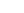. Assuming that the particle is spherical, a simple analysis to calculate critical separation particle sizes can be established.

Given that the fluid velocity is moving in a spiral the gas velocity can be broken into two component velocities: a tangential component,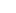, and a radial velocity component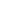. Assuming Stokes' law
Stokes' law
In 1851, George Gabriel Stokes derived an expression, now known as Stokes' law, for the frictional force – also called drag force – exerted on spherical objects with very small Reynolds numbers in a continuous viscous fluid...

, the drag force on any particle in this inlet stream is therefore given by the following equation: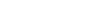If one considers an isolated particle circling in the upper cylindrical component of the cyclone at a rotational radius offrom the cyclone's central axis, the particle is therefore subjected to centrifugal, drag
Drag (physics)
In fluid dynamics, drag refers to forces which act on a solid object in the direction of the relative fluid flow velocity...

and buoyant
Buoyancy
In physics, buoyancy is a force exerted by a fluid that opposes an object's weight. In a column of fluid, pressure increases with depth as a result of the weight of the overlying fluid. Thus a column of fluid, or an object submerged in the fluid, experiences greater pressure at the bottom of the...

forces. The centrifugal component is given by: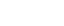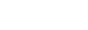The buoyant force component is obtained by the difference between the particle and fluid densities,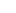and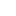respectively: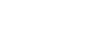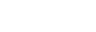The force balance can be created by summing the forces together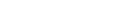This rate is controlled by the diameter of the particle's orbit around the central axis of the cyclone. A particle in the cyclonic flow will move towards either the wall of the cyclone, or the central axis of the cyclone until the drag, buoyant and centrifugal forces are balanced. Assuming that the system has reached steady state, the particles will assume a characteristic radius dependent upon the force balance. Heavier, denser particles will assume a solid flow at some larger radius than light particles. The steady state balance assumes that for all particles, the forces are equated, hence: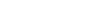Which expands to: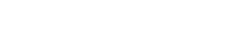This can be expressed by rearranging the above in terms of the particle radius. The particle radius as a function of cyclonic radius, fluid density and fluid tangential and rotational velocities can then be found to be: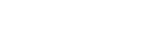Experimentally it is found that the velocity component of rotational flow is proportional to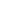, therefore: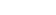This means that the established feed velocity controls the vortex rate inside the cyclone, and the velocity at an arbitrary radius is therefore: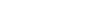Subsequently, given a value for, possibly based upon the injection angle, and a cutoff radius, a characteristic particle filtering radius can be estimated, above which particles will be removed from the gas stream.

Assume we have a particle of radius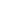and densitymoving with a parcel of fluid of viscosity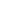and density. The particle and the fluid are moving along a curved trajectory with tangential velocitywith a radius of curvature of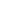.

If we view the particle in a frame of reference moving with the fluid, we can describe the behavior of the particle by invoking the imaginary, inertial centrifugal force acting as a form of gravity directed outward, away from the axis of rotation. The magnitude of the centrifugal force will be given by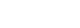.
where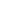is the mass of the particle.

If we ignore the universal downward force of gravity and viscous drag between the particle and the fluid parallel to the velocity, there are two other forces acting on the particle - radial viscous drag and buoyancy.

The viscous drag (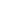) between the particle and the fluid resulting from radial movement of the particle through the fluid is given by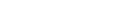whereis the radial drift velocity of the particle through the fluid and the sign reflects the opposition of the force to the motion.

The buoyancy force (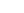) exerted on the particle by the fluid is given by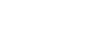where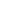is the volume of the particle

If we assign upward (toward the center of rotation) as the positive radial direction (+) in our frame of reference, then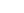will be pointed in the negative direction,will be pointed in the positive direction and the direction ofwill depend on the direction of.

If we assume the system has reached dynamic equilibrium then the sum of the forces is zero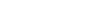.

After applying the appropriate signs and expandingandexplicitly we have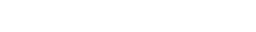Solving this equation forwe have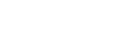.

Notice that if the density of the fluid is greater than the density of the particle, the motion is (+), toward the center of rotation and if the particle is denser than the fluid, the motion is (-), away from the center.

Expressing the motion in terms of angular velocitywe have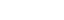Substituting into the equation above yields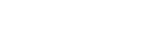.

In this analysis,is the drift velocity at which dynamic equilibrium is attained - the drag friction generated by the movement of the particle through the fluid balances the centrifugal force of the rotation and the particle has no radial acceleration, traveling at a constant velocity. In the extreme case where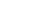(a fluid with no viscosity) the equilibrium drift velocity is undefined – the particle can accelerate without ever reaching equilibrium. In the opposite extreme,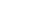∞, the equilibrium drift velocity is 0, there is no outward radial movement and the particle is frozen in the fluid

In non-equilibrium conditions, the general case equation F=ma must be solved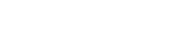The presence of both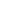and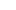makes this a differential equation and complicates the solution. Note that if the densities of the particle and fluid are equal, the solution is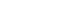and cyclonic separation is not possible.

In a cyclone particle separator, the design objective is to control the system geometry and the operating parameters so that the drift velocity will move the particle out of the carrier fluid, before the fluid exits via the outlet tube. In most cases, the steady state solution is used as guidance in designing a separator, but the actual performance must be evaluated and modified empirically.

## Alternate models

The above equations are relatively simple and provide a basic approximation to the behaviour of a cyclone separator. These equations are, however, limited in many regards. For example, the geometry of the separator is not considered, the particles are assumed to achieve a steady state and the effect of the vortex inversion at the base of the cyclone is also ignored, all behaviours which are unlikely to be achieved in a cyclone at real operating conditions.

More complex differential equation
Differential equation
A differential equation is a mathematical equation for an unknown function of one or several variables that relates the values of the function itself and its derivatives of various orders...

based models exist, as many authors have studied the behaviour of cyclone separators. Numerical modelling using computational fluid dynamics
Computational fluid dynamics
Computational fluid dynamics, usually abbreviated as CFD, is a branch of fluid mechanics that uses numerical methods and algorithms to solve and analyze problems that involve fluid flows. Computers are used to perform the calculations required to simulate the interaction of liquids and gases with...

has also been used extensively in the study of cyclonic behaviour.